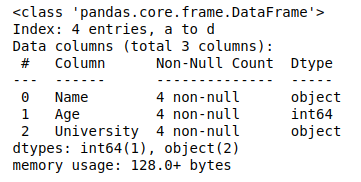# Get the number of rows and number of columns in Pandas Dataframe

Pandas provide data analysts a variety of pre-defined functions to Get the number of rows and columns in a data frame. In this article, we will learn about the syntax and implementation of few such functions.

#### Method 1: Using df.axes() Method

`axes()` method in pandas allows to get the number of rows and columns in a go. It accepts the argument ‘0’ for rows and ‘1’ for columns.

Syntax: df.axes[0 or 1]

Parameters:
0: for number of Rows
1: for number of columns

Example:

 `# import pandas library ` `import` `pandas as pd ` `   `  `# dictionary with list object in values ` `details ``=` `{ ` `    ``'Name'` `: [``'Ankit'``, ``'Aishwarya'``, ``'Shaurya'``, ``'Shivangi'``], ` `    ``'Age'` `: [``23``, ``21``, ``22``, ``21``], ` `    ``'University'` `: [``'BHU'``, ``'JNU'``, ``'DU'``, ``'BHU'``], ` `} ` `   `  `# creating a Dataframe object  ` `df ``=` `pd.DataFrame(details, columns ``=` `[``'Name'``, ``'Age'``, ``'University'``],\ ` `                  ``index ``=` `[``'a'``, ``'b'``, ``'c'``, ``'d'``]) ` `   `  `# Get the number of rows and columns ` `rows ``=` `len``(df.axes[``0``]) ` `cols ``=` `len``(df.axes[``1``]) ` ` `  `# Print the number of rows and columns ` `print``(``"Number of Rows: "` `+` `str``(rows)) ` `print``(``"Number of Columns: "` `+` `str``(cols)) `

Output:

```Number of Rows: 4
Number of Columns: 3
```

#### Method 2: Using df.info() Method

`df.info()` method provides all the information about the data frame, including the number of rows and columns.

Syntax:

`df.info`

Example:

 `# import pandas library ` `import` `pandas as pd ` `   `  `# dictionary with list object in values ` `details ``=` `{ ` `    ``'Name'` `: [``'Ankit'``, ``'Aishwarya'``, ``'Shaurya'``, ``'Shivangi'``], ` `    ``'Age'` `: [``23``, ``21``, ``22``, ``21``], ` `    ``'University'` `: [``'BHU'``, ``'JNU'``, ``'DU'``, ``'BHU'``], ` `} ` `   `  `# creating a Dataframe object  ` `df ``=` `pd.DataFrame(details, columns ``=` `[``'Name'``, ``'Age'``, ``'University'``], ` `                  ``index ``=` `[``'a'``, ``'b'``, ``'c'``, ``'d'``]) ` `   `  `# Get the info of data frame ` `df.info() `

Output:Here in the above code, the value in the Index gives the number of rows and the value in Data columns gives the number of columns.

#### Method 3: Using len() Method

`len()` method is used to get the number of rows and number of columns individually.

Syntax:

```len(df)
and
len(df.columns)```

Example 1: Get the number of rows

 `# import pandas library ` `import` `pandas as pd ` `   `  `# dictionary with list object in values ` `details ``=` `{ ` `    ``'Name'` `: [``'Ankit'``, ``'Aishwarya'``, ``'Shaurya'``, ``'Shivangi'``], ` `    ``'Age'` `: [``23``, ``21``, ``22``, ``21``], ` `    ``'University'` `: [``'BHU'``, ``'JNU'``, ``'DU'``, ``'BHU'``], ` `} ` `   `  `# creating a Dataframe object  ` `df ``=` `pd.DataFrame(details, columns ``=` `[``'Name'``, ``'Age'``, ``'University'``], ` `                  ``index ``=` `[``'a'``, ``'b'``, ``'c'``, ``'d'``]) ` `   `  `# Get the number of rows ` `print``(``"Number of Rows:"``, ``len``(df)) `

Output:

```Number of Rows: 4
```

Example 2: Get the number of columns

 `# import pandas library ` `import` `pandas as pd ` `   `  `# dictionary with list object in values ` `details ``=` `{ ` `    ``'Name'` `: [``'Ankit'``, ``'Aishwarya'``, ``'Shaurya'``, ``'Shivangi'``], ` `    ``'Age'` `: [``23``, ``21``, ``22``, ``21``], ` `    ``'University'` `: [``'BHU'``, ``'JNU'``, ``'DU'``, ``'BHU'``], ` `} ` `   `  `# creating a Dataframe object  ` `df ``=` `pd.DataFrame(details, columns ``=` `[``'Name'``, ``'Age'``, ``'University'``], ` `                  ``index ``=` `[``'a'``, ``'b'``, ``'c'``, ``'d'``]) ` `   `  `# Get the number of columns ` `print``(``"Number of Columns:"``, ``len``(df.columns)) `

Output:

```Number of Columns: 3
```

#### Method 4: Using df.shape() Method

`df.shape()` method returns the number of rows and columns in the form of a tuple.

Example:

 `# import pandas library ` `import` `pandas as pd ` `   `  `# dictionary with list object in values ` `details ``=` `{ ` `    ``'Name'` `: [``'Ankit'``, ``'Aishwarya'``, ``'Shaurya'``, ``'Shivangi'``], ` `    ``'Age'` `: [``23``, ``21``, ``22``, ``21``], ` `    ``'University'` `: [``'BHU'``, ``'JNU'``, ``'DU'``, ``'BHU'``], ` `} ` `   `  `# creating a Dataframe object  ` `df ``=` `pd.DataFrame(details, columns ``=` `[``'Name'``, ``'Age'``, ``'University'``], ` `                  ``index ``=` `[``'a'``, ``'b'``, ``'c'``, ``'d'``]) ` `   `  `# Get the number of Rows and columns ` `df.shape `

Output:

`(4, 3)`

Attention geek! Strengthen your foundations with the Python Programming Foundation Course and learn the basics.

To begin with, your interview preparations Enhance your Data Structures concepts with the Python DS Course.

My Personal Notes arrow_drop_upCheck out this Author's contributed articles.

If you like GeeksforGeeks and would like to contribute, you can also write an article using contribute.geeksforgeeks.org or mail your article to contribute@geeksforgeeks.org. See your article appearing on the GeeksforGeeks main page and help other Geeks.

Please Improve this article if you find anything incorrect by clicking on the "Improve Article" button below.

Article Tags :

Be the First to upvote.

Please write to us at contribute@geeksforgeeks.org to report any issue with the above content.